# What is Frequency?

What is Frequency?

Frequency, also known as the number of vibrations; is formed by the regular repetition of an event, movement in a certain time interval. To measure the frequency, repetitive movements are calculated at equal time intervals. In other words, the frequency can be explained as “the number of repetitions of a movement per unit time“. The time interval in which the movement is repeated is called a period. It is denoted by Period T. The frequency unit is shown in Hertz (Hz).

Waves that repeat within 1 second are called frequency. For example; If the time it takes for a wave to pass is seconds, the frequency is measured as 2 per second.

The wavelength of the frequency is measured by a device called an Oscilloscope. The frequency was discovered in the 19th century by the German physicist Heinrich Rudolf Hertz, and hence the frequency unit is denoted as Hertz.

## How is Frequency Measured?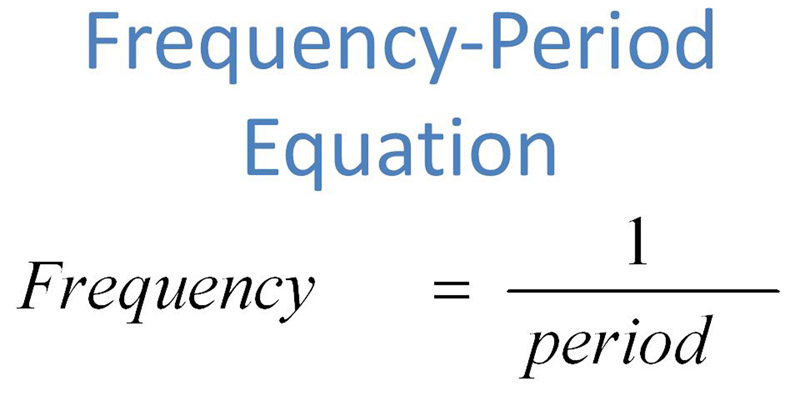In this way, the frequency measurement formula is created. The formula used for frequency measurement is as follows;

F = 1 / T

Frequency can be measured using this formula. Terms such as megahertz (MHz) or kilohertz (kHz) can be used to describe very large frequencies.

### What are the Types of Frequency?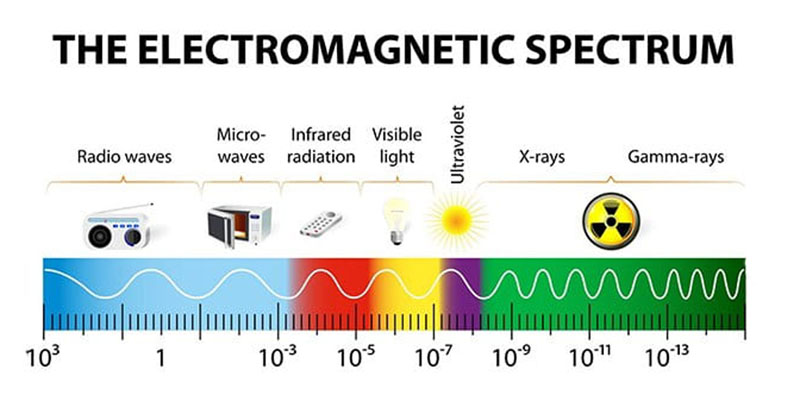Frequency is a term related to many different topics. At this point, the frequency types are as follows;

1. Radio Frequency: It is the type of frequency used in radio frequency communication technology known as electromagnetic waves and in which wireless signals are followed. The radiofrequency occurs between 30 and 300 kHz.
2. Light Frequency: It is the frequency type that expresses the color of the light. At this point, the high spectrum light frequency is expressed in blue, and the low spectrum light frequency in red.
3. Sound Frequency: It is the frequency type that expresses the pressure change within one second. The human voice can hear sound waves between 15 HZ and 20 kHz. Sound Frequency is measured in Decibels (Db).

Frequency Ranges: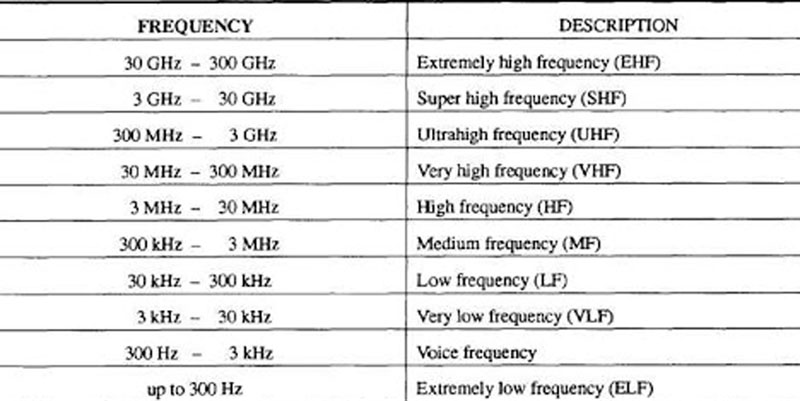• Low Frequency: It ranges from 300 kHz to 3 MHz. (Infrasonic)
• Medium Frequency: It is in the range of 3 to 30 MHz.
• High Frequency: Between 30 and 300 MHz. (Ultrasonic)

As Hatko Sound Barrier, we know that loud noise, in other words, has harmful effects on human health. That’s why we apply sound barriers or noise barriers to areas with high sound frequency. We aim to reduce loud noise, which has a high frequency such as traffic noise and causes deep damage to human psychology, thanks to the noise barrier, which is usually built on the side of the highway.

Therefore, we would like to give you detailed information about the sound frequency.

## What is Sound Frequency?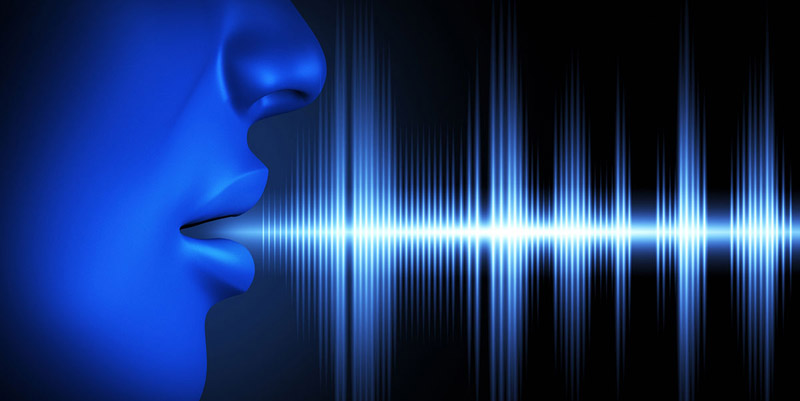There are sounds that the human ear can and cannot perceive. For example, while the human ear can perceive sounds between 20 and 20000 hertz, it cannot perceive sounds that are smaller than 20 and greater than 20000.

Each country has its own unique sound value. For example, this value Turkey alternating current at 50 Hz to 60 Hz as measured while in the United States.

Sound is actually a type of energy and it occurs when vibrations turn into energy. Sound is measured in Decibels and the strength of the sound is called loudness.

The most important substance necessary for the propagation of sound is air. Therefore, sound can’t spread outside the atmosphere. In other words, the sound does not spread in space. Sound propagates at different frequencies in different substances. For example, sound waves propagate differently in solid, liquid, and gaseous environments.

In solids; Sound propagating at 5000 m / s in water; It spreads at a velocity of 1453 m / s and 340 m / s in the air.

### What Are the Sources of Sound?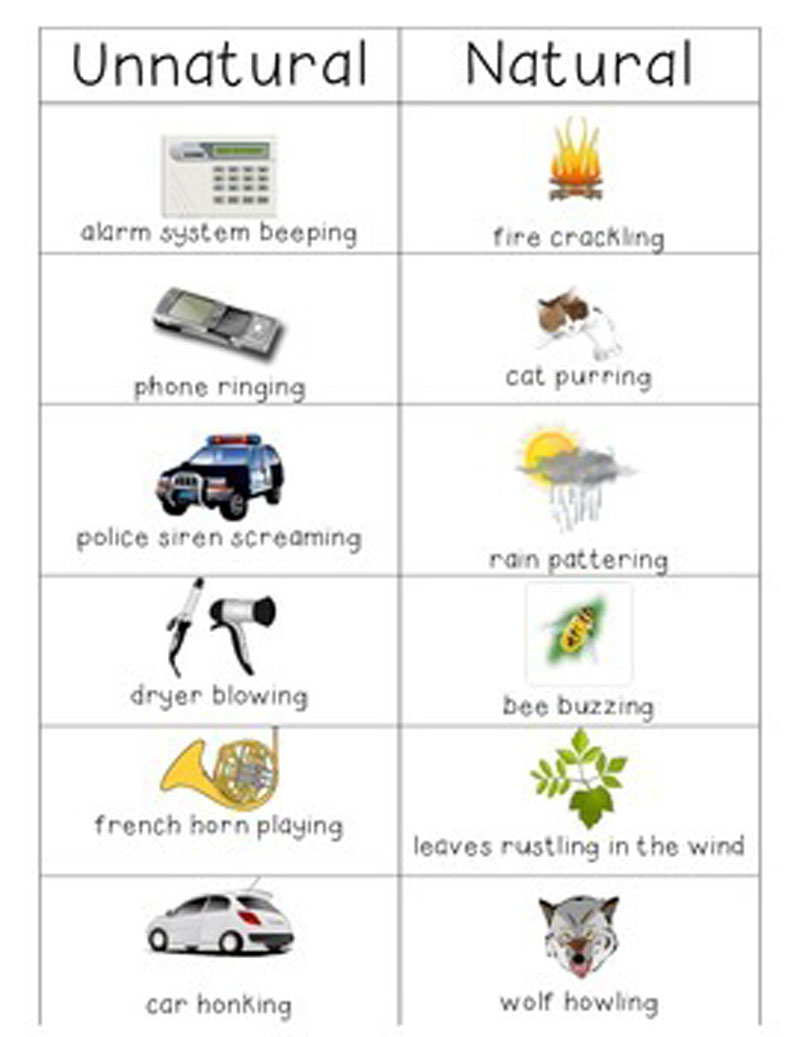Sound sources are divided into 2.

1. Natural Sound Sources

Natural sound sources are the names given to sounds that occur spontaneously in nature. For example, sounds such as rain, water, wind, thunder, and animal sounds are natural sound sources.

1. Artificial Sound Sources

Sound sources created by humans are called artificial sound sources. For example, sounds such as car sound, telephone sound, musical instrument sound are among the artificial sound sources.

Some of these artificial sound sources created by humans can be harmful to humans. For example, sounds that have a very high sound frequency and we call noise harm people. Today, many methods have been developed to prevent noise. While sound insulation systems were created for houses, sound barriers were created for highways.

The noise generated by the sound barrier, that is, the noise barrier, is ensured to be trapped in that area and the spread of the noise into the city is prevented.

In this way, the harmful factors created by artificial sound sources do not harm people. As Hatko Sound Barrier, we have been working for many years to reduce the noise of city life. For more information about the sound barriers, you can check our website and contact us.